# Precalculus : Pre-Calculus

## Example Questions

### Example Question #1 : Symmetry

If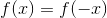, what kind of symmetry does the functionhave?

Possible Answers:

Even Symmetry

Symmetry across the line y=x

Odd Symmetry

No Symmetry

Correct answer:

Even Symmetry

Explanation:

The definition of even symmetry is if### Example Question #7 : Graphing Functions

If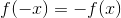, what kind of symmetry doeshave?

Possible Answers:

No symmetry

Even symmetry

Symmetry across the line y=x

Odd symmetry

Correct answer:

Odd symmetry

Explanation:is the definition of odd symmetry

### Example Question #1 : Rational Functions

Determine the equation(s) of the vertical asymptote(s) of the following rational equation: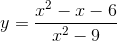Possible Answers: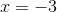There are no vertical asymptotes for this equation.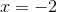Correct answer:Explanation:

We recall that a vertical asymptote of a rational function is found whenever the factors of the denominator equal 0, provided that there are no cancelling factors in the numerator. We have to factor both the numerator and the denominator.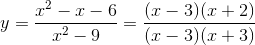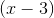cancels, so there is a hole in our graph at.

The remaining factor in the denominator can be set equal to 0 to find the asymptote: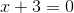### Example Question #2 : Rational Functions

Identify the equation(s) of the hole or holes (also called removable discontinuities) in the following rational equation: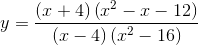Possible Answers: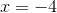There are no holes/removable discontinuities in this equation.Correct answer:Explanation:

In order to have a hole/removable discontinuity, the numerator and the denominator must share a factor - and, that factor must not appear a second time in the denominator. If it does appear a second time in the denominator, then the zero for that factor will still create a vertical asymptote, not a hole.

To find out what will happen with this equation, we factor the top and bottom completely: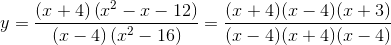Let's compare factors now: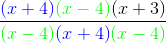The removable discontinuity must be at.

### Example Question #3 : Rational Functions

Determine the equations of all asymptotes of the following rational equation: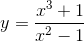Possible Answers:

Vertical asymptote:Slant asymptote: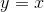Vertical asymptote: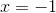Slant asymptote:Vertical asymptote:Slant asymptote: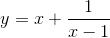Vertical asymptote: None

Horizontal asymptote: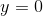Vertical asymptotes: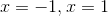Slant asymptote: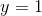Correct answer:

Vertical asymptote:Slant asymptote:Explanation:

First, in order to find vertical asymptotes, we factor the top and bottom and look for factors in the denominator which do not cancel with factors in the numerator.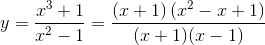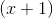cancels, leaving only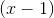in the denominator.

Therefore, there is a vertical asymptote at.

This leaves us to look for the horizontal/slant asymptote. We know that it will be a slant asymptote because the numerator is of a higher degree than the denominator. To find a slant asymptote, we divide the factors which did not cancel and consider the quotient.

We can disregard the remainder, so there is no need to do fully long devision here. We are left with a slant asymptote of.

### Example Question #4 : Rational Functions

Vertical asymptotes.

Find the vertical asymptote of the function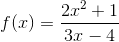Possible Answers: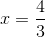No asymptote.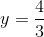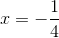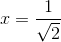Correct answer:Explanation:

Vertical asymptotes occur when the function values increase without bound as the input approaches a certain value.  In other words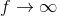for some value of x, which is the line describing the vertical asymptote.

Now, anytime a denominator is 0, the value of a fraction or rational function is undefined.  This is where.

The denominator in this function is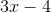So, solve for x in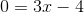To get.

### Example Question #5 : Rational Functions

Oblique asymtotes.

Find the oblique, or slant asymptote of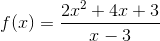.

Possible Answers: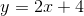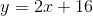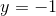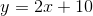Correct answer:Explanation:

When the degree of the numerator is greater than the degree of the denominator by a factor of 1, then a function will have an oblique asymptote.  The numerator increases faster than the numerator by a factor of x, so there will be a line with a slope that the graph will approach but not intersect.

The asymptote can be found by performing polynomial or synthetic division, and ignoring the remainder.

Synthetic division of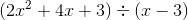gives

3_|  2   4    3

6   30

_______

2  10 |_33

The quotient witout the remainder is the asymptote.

### Example Question #6 : Rational Functions

Determine the vertical asymptotes of the following equation: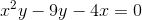Possible Answers: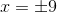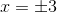There are no vertical asymptotes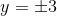Correct answer:Explanation:

First, start by isolating x and y on seperate sides to get the equation into the form of y=x. Do this by adding 4x to both sides.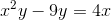Next, factor out a y on the right side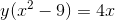Divide both sides by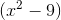to get in the for of y=x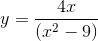Now that the equation is in the y=x form, we can set the denominator equal to 0 and solve for x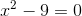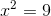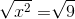### Example Question #7 : Rational Functions

Horizontal asymptotes.

Find the horizontal asymptote of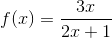.

Possible Answers: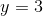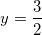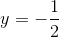Correct answer:Explanation:

A horizontal asymptote occurs when y approaches a certain value as x goes to infinity or negative infinity.  This happens in a rational expression when the degrees of the numerator and denominator are the same.  The independent variable of both terms increases or decreases at the same rate, while the terms themselves change according to their leading coefficients.   This is to say that there is a line defined by the ratio of leading coefficients that the graph will approach but not inetrsect as x increases or decreases indefinely.

The leading coeffiecint of the numerator is 3, while the leading coefficient of the denominator is 2.  So the asymptote is the line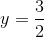.

### Example Question #8 : Rational Functions

What is the horizontal asymptote for the function below?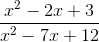Possible Answers: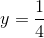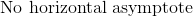Correct answer:Explanation:

Since the leading terms of the numerator and the denominator have the same degree, the ratio will move towards a constant.  The ratio of the leading coefficients is 1/1, so the function will taper off to y = 1 in both the positive and negative directions for x.

### All Precalculus Resources